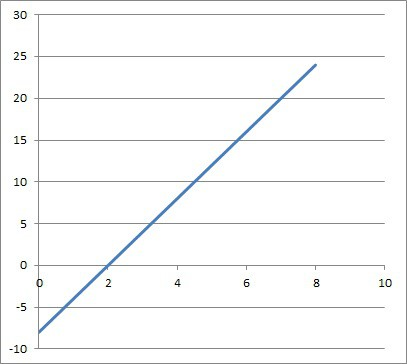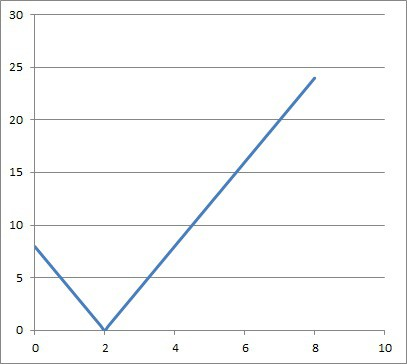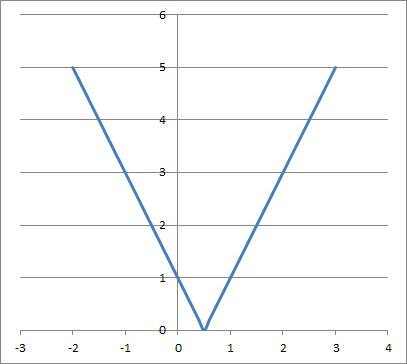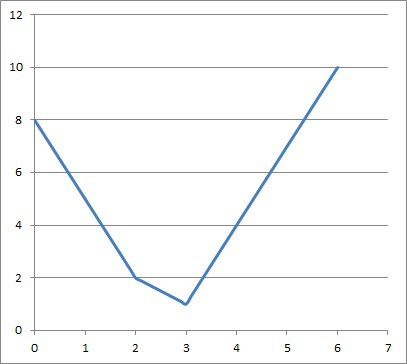# When To Use Absolute Value (3 Things To Know)

Absolute value is used throughout mathematics and in other disciplines as well, including physics and finance.  However, it still helps to know exactly when it might be used, along with how to solve the equations or inequalities that arise.

So, when do you use absolute value? Absolute value is used to express the distance between two numbers on a number line. It is also used to find the speed of an object and the distance it travels over time. Absolute value can express a range of values, and we can use it to compute percent error.

Of course, absolute value can never be negative, so we would not use it if we want to know whether an estimate is above or below the actual value.

In this article, we’ll talk about absolute value and when to use it.  We’ll also look at some examples of how to solve absolute value equations and inequalities.

Let’s get started.

## When To Use Absolute Value

Absolute value has many uses in mathematics and in various disciplines, including:

• Distance from zero on a number line: |x| = |x – 0| is the distance from zero to x (in units).
• Distance between two points on a number line: |x – y| is the distance from x to y (in units).
• Speed of an object: graph the absolute value of velocity over time to get the graph of speed.
• Distance traveled by an object: graph the absolute value of velocity over time, then find the total area under curve and above the x-axis to get the distance.
• Range of Values: |x| <= 4 is compact way to express the range of value -4 <= x <= 4 or the interval [-4, 4].
• Error: comparing actual vs. expected values, or to see how far off we are, without regard to sign (absolute error or percent error).

Note: in Excel or Google sheets, the notation for the absolute value function is =ABS(x), where x is a number.

### Absolute Value For Distance On A Number Line

We can think of absolute value as a distance function with two inputs, given by:

• D(x, y) = |x – y| = distance between x and y on a number line

If x and y have the same value (x = y), then the distance between them is zero, since:

• D(x, y) = D(x, x) = |x – x| = 0

Also, if y = 0, then the distance function is simply the absolute value of x, since:

• D(x, y) = D(x, 0) = |x – 0| = |x|

### Absolute Value For Distance & Speed Of An Object

We can also use absolute value to find an object’s speed and the distance it has traveled in a time interval.

First, remember that speed is the absolute value of velocity (unsigned velocity).  That is, speed tells us how fast an object is moving, without regard to the direction of the movement.

The graphs below show velocity and speed of an object.The velocity of an object can be negative, indicating that it is moving backwards or in a negative direction.The speed of an object cannot be negative, since speed is the absolute value of velocity (or unsigned velocity).

To find displacement of an object, we find the area between the velocity curve and the x-axis.  We count areas above the x-axis as positive, and areas below the x-axis as negative.

So, an object could have zero displacement if it moves 10 feet north from its starting position and then moves 10 feet south back to the starting position (10 + -10 = 0 feet of displacement).

However, the distance traveled would be |10| + |-10| = 10 + 10 = 20 feet.  We can also see this by finding the area under the speed curve.

### Absolute Value For A Range Of Values

If we wish to express a range of values (interval), we can use absolute value instead of a compound inequality.  For example, we can use |x| <= 4 instead of -4 <= x <= 4 or, as an interval, [-4, 4].

The general formula for the range of values C – R <= x <= C + R is:

• |x – C| <= R

where C is the center of the interval and R is the “radius” (half the width of the interval).

If the interval is [a, b], then we have:

• C = (a + b) / 2  [the center of an interval is the average of the endpoints]
• R = (b – a) / 2  [the radius of an interval is half of the width of the interval]

#### Example: Express A Range Of Values Using Absolute Value

Let’s say we want to express the interval [-6, 10] or -6 <= x <= 10 using absolute values.  Then we have:

• C = (a + b) / 2  = (10 + -6) / 2 = 4 / 2 = 2
• R = (b – a) / 2 = (10 – (-6)) / 2 = 16 / 2 = 8

So, the center of the interval is 2, and the radius is 8 (the width is 16).

The inequality is:

• |x – C| <= R
• |x – 2| <= 8

We can check this by solving:

• |x – 2| <= 8
• -8 <= x – 2 <= 8
• -8 + 2 <= x <= 8 + 2
• -6 <= x <= 10

### Absolute Value For Error

We use absolute value when we want to find out how much an actual value differs from an expected value, without regard to sign.

For example, let’s say we are predicting the sale price of houses that go up for sale in a neighborhood.  The following table shows the actual values (sales prices), our predicted values (expected), the differences (absolute value), and the percent error.

Note: in some cases, it will matter whether the actual value is above or below the expected value.  In these cases, it is not appropriate to use absolute value.

For example, let’s say we want to find out if our estimates are high or low.  If we often or always get a negative value for actual minus expected, then our home price predictions are too high.

In that case, we would want to revise our projections downward.

## How Do You Know When To Use Absolute Value?

The wording in a problem often gives us context and indicates when to use absolute value.  The following vocabulary can tell us when to use absolute value:

• Distance
• Speed
• Range
• Error (Actual vs Expected)
• Percent Error

## How Do You Solve Problems With Absolute Value?

Solving problems with absolute value often involves two separates “halves”: the positive half and the negative half.  For example, there are two solutions to |x| = 2: x = +2 and x = -2.

This means that an absolute value that is equal to a positive number will be an equation with two solutions.

Let’s take a look at how to solve absolute value problems, starting with equations.

### Solving Absolute Value Equations

Solving absolute value equations usually involves two halves: the positive and negative halves.  However, there are some special cases where there is only one half (when the absolute value is equal to zero).

There are also cases when there is no solution (when the absolute value is equal to a negative number).  Let’s see some examples.

#### Example 1: Solving An Absolute Value Equation With Two Solutions

Consider the absolute value equation

• |x – 2| = 5

Since |-5| = 5 and |5| = 5, there are two “halves”, or solutions:

x – 2 = -5 and x – 2 = 5

x = -3 and x = 7

#### Example 2: Solving An Absolute Value Equation With One Solution

Consider the absolute value equation

• |x + 4| = 0

Since only |0| = 0, there is only one solution:

• x + 4 = 0
• x = -4

#### Example 3: An Absolute Value Equation With No Solution

Consider the absolute value equation

• |x – 1| = -7

Since an absolute value is always nonnegative (either positive or zero), there is no way that an absolute value can be negative.  So, there is no solution to this equation.

### Solving Absolute Value Inequalities

Solving absolute value inequalities usually involves two halves: the positive and negative halves.  However, there are also cases when there is no solution.  Let’s see some examples.

#### Example 1: Solving An Absolute Value Inequality With Two “Halves”

Consider the absolute value inequality

• |x – 3| <= 4

We have to consider two halves for the solution simultaneously:

• -4 <= x – 3 <= 4
• -4 + 3 <= x – 3 + 3 <= 4 + 3
• -1 <= x <= 7

So, the solution is the interval [-1, 7].

#### Example 2: Solving An Absolute Value Inequality With Two “Halves”

Consider the absolute value inequality

• |x + 1| >= 6

We have to consider two halves for the solution simultaneously:

• x + 1 >= 6 or x + 1 <= -6
• x + 1 – 1 >= 6 – 1 or x + 1 – 1 <= -6 – 1
• x >= 5 or x <= -7

So the solution is the compound inequality x >= 5 or x <= -7.

#### Example 3: Absolute Value Inequality With No Solution

Consider the absolute value inequality

• |x – 3| <= -2

Since an absolute value is always nonnegative, there is no way that the left side of this equation (an absolute value) can be less than -2.

So, there is no solution.

### Graphing Absolute Value Functions

Graphing absolute value functions is easier after converting to one or more piecewise functions and graphing from there.

#### Example 1: Graphing An Absolute Value Function

Consider the absolute value function f(x) = |x|.

As a piecewise function, we have:

• +x for x >= 0
• -x for x < 0

The graph is pictured below.

Note that this graph is always on or above the x-axis.

#### Example 2: Graphing An Absolute Value Function

Consider the absolute value function f(x) = |2x – 1|.

As a piecewise function, we have:

• 2x – 1 for x >= 1/2
• 1 – 2x for x < 1/2

The graph is pictured below.The absolute value of 2x – 1 is never negative. We can write it as a piecewise function if we wish.

Note that this graph is always on or above the x-axis.

### How Do You Solve Absolute Value Equations With Two Absolute Values?

To solve an absolute value equation with two (or more) absolute values, convert the absolute value functions to piecewise functions.  Then, combine them, and graph the resulting “master” piecewise function to see where the solutions lie.

#### Example: Graphing An Absolute Value Equation With Two Absolute Values

Consider the absolute value equation

• |x – 2| + |2x – 6| = 4

For the first part, |x – 2|, the piecewise graph is:

• x – 2, x >= 2
• 2 – x, x < 2

Its graph is pictured below:

For the second part, |2x – 6|, the piecewise graph is:

• 2x – 6, x >= 3
• 6 – 2x, x < 3

Its graph is pictured below:

Taking the sum of these two, we get:

• 8 – 3x, x < 2
• 4 – x, 2 <= x < 3
• 3x – 8, x >= 3

The graph of this function is pictured below.The graph of |x – 2| + |2x – 6| is piecewise, and changes at x = 2 and x = 3.

We can see where this graph intersects the line y = 4 by solving the first part:

• 8 – 3x = 4, x < 2
• -3x = -4, x < 2
• x = 4/3, x < 2
• x = 4/3

the second part:

• 4 – x = 4, 2 <= x < 3
• – x = 4, 2 <= x < 3
• x = -4, 2 <= x < 3
• No solution

and the third part:

• 3x – 8 = 4, x >= 3
• 3x = 12, x >= 3
• x = 4, x >= 3
• x = 4

So, the solutions are x = 4/3 and x = 4, as we can verify from the graph.

## Conclusion

Now you know when to use absolute value and how to solve the resulting equations and inequalities.  You also know when these equations and inequalities have no solutions.